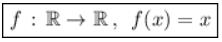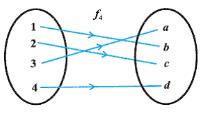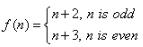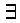Courses

# Test: Introduction To Functions

## 15 Questions MCQ Test Mathematics (Maths) Class 12 | Test: Introduction To Functions

Description
This mock test of Test: Introduction To Functions for JEE helps you for every JEE entrance exam. This contains 15 Multiple Choice Questions for JEE Test: Introduction To Functions (mcq) to study with solutions a complete question bank. The solved questions answers in this Test: Introduction To Functions quiz give you a good mix of easy questions and tough questions. JEE students definitely take this Test: Introduction To Functions exercise for a better result in the exam. You can find other Test: Introduction To Functions extra questions, long questions & short questions for JEE on EduRev as well by searching above.
QUESTION: 1

### If y is expressed in terms of a variable x as Y = ƒ(x), then y is called

Solution:

If y is expressed in terms of a variable x as y=f(x), then y is called explicit function.

QUESTION: 2

### Let f : R → R defined as f(x) = x be an identity function. Then

Solution:

Here, we are given a function f ,which is defined from R to R. Also, f is said to be the Identity Function.
The Identity Function returns the same output as given in the input.
That is, if you input some x into f(x), you get the result as x itself. So, the Identity Function is:As we can see, the Domain of the function is R , and the co-domain as well as range is also R.

QUESTION: 3

### Let A = {1, 2, 3} and B = {5, 6, 7, 8, 9} and let f(x) = {(1, 8), (2, 7), (3, 6)} then f is

Solution:
QUESTION: 4In the mapping above, the function f4 is an:

Solution:

Clearly, mappings given in options (a),(b) and (c ) satisfy the given conditions and are one-one onto.

QUESTION: 5

Let f : N →N be defined byfor all n ∈ N.
Then, the function f is

Solution:

F(n) = n+2 if n is odd
so if n=1, then f(1)= 3
if n=3 , f(3)=5
On the other hand
F(n)= n+3 if n is even
if n= 2,f(2)=5
if n=4 ,f(4)= 7
Clearly f(3) = f(2) = 5
but 3 does not equals to 3, so it is not one one i.e. injective also 1€N
i.e. codomain
but here 1€ range does not equals to codomain so it is not surjective.

QUESTION: 6

A function f: R → R defined as f(x) = x4 is​

Solution:

f: R → R is defined as f(x) = x4
Let x, y ∈ R such that f(x) = f(y).
=> x4 = y4
=> x =+-y
Therefore, f(x1) = f(x2) does not imply that x1=x2.
For instance,f(1) = f(-1) = 1
∴ f is not one-one.
Consider an element 2 in co-domain R. It is clear that there does not exist any x in domain R such that f(x) = 2.
∴ f is not onto.
Hence, function f is neither one-one nor onto.

QUESTION: 7

A function f: X → Y is bijective if and only if​

Solution:
QUESTION: 8

A function f: X → Y is injective if and only if

Solution:

Let X, Y be sets, and let f : X → Y be a function. We say that f is injective (sometimes called one-to-one) if ∀x1, x2 ∈ X, f(x1) = f(x2
⇒ x1 = x2.

QUESTION: 9

A function f: R → R defined as f(x) = 5x is​

Solution:

Suppose x1 and x2 are real numbers such that f(x1) = f(x2). (We need to show x1 = x2 .)
5x1  = 5x2
Dividing by 5 on both sides gives
x1 = x2  (function is one - one)
Let y ∈R. (We need to show thatx in R such that f(x) = y.)
If such a real number x exists, then 5x -2 = y and x = (y + 2)/5. x is a real number since sums and quotients (except for division by 0) of real numbers are real numbers. It follows that
f(x) = 5((y + 2)/5)
by the substitution and the definition of f
= y + 2
= y
by basic algebra
Hence, f is onto.

QUESTION: 10

A function f: X → Y is surjective if and only if​

Solution:

A function f (from set X to Y) is surjective if and only if for every y in Y, there is at least one x in X such that f(x) = y, in other words f is surjective if and only if f(X) = Y.

QUESTION: 11

The range of function f : R → R defined by f(x) = x2 is​

Solution:

As x is square of every real number, so the f(x) = x2 cannot be the negative.

QUESTION: 12

Let f : R → R defined as f(x) = 5 be a constant function. Then​

Solution:
QUESTION: 13

The function, f(x) = 2x + 1 is

Solution:

Let the domain of x be R
For surjectivity-
Let f(x) = Y
=> 2x +1 = Y
=> x = (Y - 1)/2
It is clear that Y takes all the values from (-∞, +∞)
So the range of f(x) is the same as the domain of x.
So it is surjective.

QUESTION: 14

Let f: {1, 2, 3} → {1, 2, 3} be an onto function. Then, f is​

Solution:

(i) f(1)=1,f(2)=2,f(3)=3
(ii) f(1)=1,f(2)=3,f(3)=2
(iii) f(1)=2,f(2)=3,f(3)=1
(iv) f(1)=2,f(2)=1,f(3)=3
(v) f(1)=3,f(2)=1,f(3)=2
(vi) f(1)=3,f(2)=2,f(3)=1
Since, f is onto, all elements of {1,2,3} have unique pre-image.
The above cases are possible.
Since, every element 1,2,3 has either of image 1,2,3 and that image is unique.
∴  f is one-one.
∴ Function f:A→A is one-one.

QUESTION: 15

A function f: Z → Z defined as f(x) = x3 is​

Solution:

f(x) = x3
Checking one-one f(x1) = (x1)3
f(x2) = (x2)3
Comparing f(x1) = f(x2)
(x1)3 = (x2)3
x1 = x2   => x1 = -x2
Since, x1 & x2 are natural number, they are always positive.
Hence, x1 = x2  ( it is one - one)
Checking onto : f(x) = (x)3
Let f(x) = y such that y implies N
x3 = y
x = (y)^⅓
x = 1.71, hence it is not a natural number
Thus function is not onto